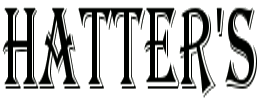CONE AND CYLINDER

All comments welcome; and, welcome as additions to the site:
hatterscabinet@gmail.com

Unless otherwise stated,
all content © A.E.M. Baumann

Cone and Cylinder: A Mathematical Aporia

– March 11, 2014

This is a little something that got into my head for a while a month back and am finally getting around to putting it up here.

Here's a little geometric image I worked up.The top image is the bisection at the origin of a cylinder. At every possible bisection at the origin, this is the resulting shape, a rectangle of area that is the product of the height (h) and the length, which is twice the radius):

A = 2rh

The volume of a cylinder is the area of the base circle times the height:

V = πr2h

Just as the area of a cube (or other rectangular prisms) is the area of the base times the height.

The bottom image is the bisection at the origin of a cone of the same height and radius. At every possible bisection at the origin, the full three hundred and sixty degrees (to mix systems), this is the bisection. Its area is made of two triangles, each of which is one half the area of the squares (which is here rh). Thus, the area of the bisection of the cone is one half the area of the bisection of the cylinder:

A = 2rh/2 = rh

For the full circle, no matter where you slice it through the origin, covering the whole area of the base, the bisection of a cone is one half of the bisection of the cylinder.

But the volume of a cone is not one-half of the volume of a cylinder of the same height and radius: which would be

πr2h/2

Rather, the volume of a cone is one-third the volume of a cylinder:

πr2h/3

So even though the bisections cover the whole of the cylinder and cone, that is, even though no matter where you slice the cone (so long as through the origin) the area of the bisection of the cone is one half the area of the bisection of the cylinder, the volume of a cone is one-sixth short. Where did that 1/6th go?

The answer lies not in the geometry but within the question itself; that is, in the assumptions (the aprioris) that give meaning to the question. What is going on here is another demonstration of how math systems (and the sciences that build upon them) are wholly abstract: they are not built upon empirical reality but only entirely upon themselves. That is, there is no such thing as the number 2 in nature. It is, to use Bruno against how the scientific rationalists want to use him, a presentation of hieroglyphic understanding; in Coleridge's terms, symbolic understanding. It is of the nature of da Cusa's deconstructions of geometry, as with "a circle of infinite radius is a straight line."

(Though, I admit, of a different sort, and not as graceful a one.)